# Introduction

When creating a colour scheme for a website, one method is to use different quantities of red, green and blue to create the perfect colour. On geogebra, we can select colours by choosing the ratio of red, green, blue. Here is a screenshot: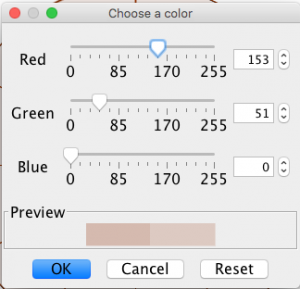Notice that there are 256 parts of each colour (include the ‘0’). Its important in this project to know that.

Here is a screen shot of the brown wheel we begin with in this project.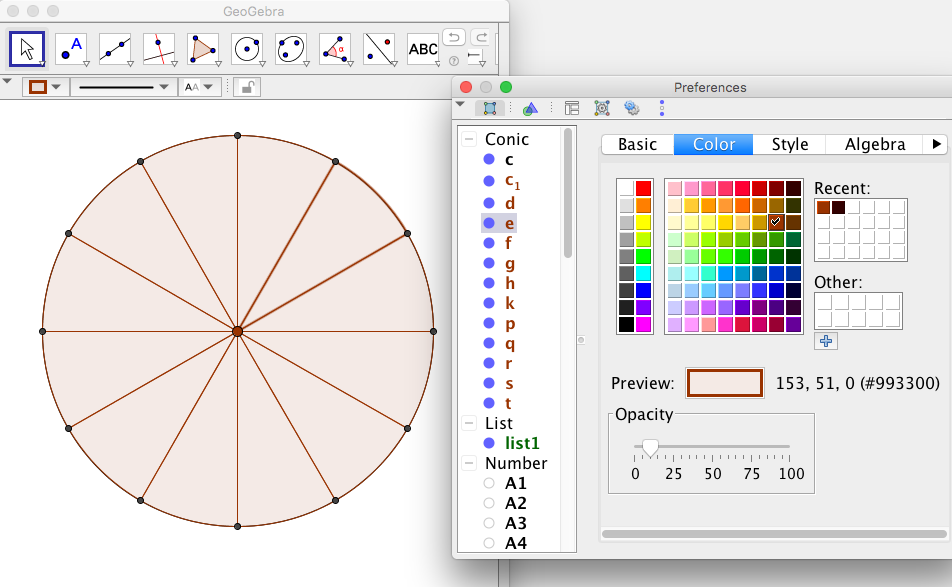Notice, the default brown colour is made up of 153 Red, 51 Green, 0 Blue and has the html name #993300. The html name is made up of three hexadecimal values.

# The Project

As you can see from the screenshots above, colours in geogebra are input using red, green, blue ratios; however the hexadecimal name is also given.

There are four steps to the project:

• First, let’s do the colouring, and take note of the colours used in hexadecimal code (the number in brackets that begins with a #).You can print out Colour Wheel Notes to record your colours. Use at least three different colours in your design. Take a screenshot to save your design.
• Next, we’ll discuss how to understand and to write numbers in different bases.
• Translate the hexadecimal code given to you and reproduce your partner’s design.

Here’s the applet:

Remember to take a screen shot to save your colour wheel (on Mac, use command shift 4; on PC, search for the ‘snipping tool’). Now put your colour wheel aside and do the following research on number bases. We’ll get back to your colour wheel at the end of this page.

# Basics – writing numbers in base 10

Thanks to the Chinese, Hindu and Arabic mathematicians of ancient history, we generally name our numbers after powers of 10. One thousand is. One hundred is. We count in these powers, however we are so familiar with them we don’t really notice it.

Take the number– three thousand, four hundred and fifty seven. We can write it as:or,We multiply the powers of ten with only the digits. After we reach the number 9, we move into a new power of 10.

As another example, the numbercan be written as:Notice the use of(which we know is equal to one) to write the ‘units’ and to maintain the pattern.

## Short Comprehension Exercise:

1. Write the number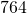in the form.
2. Write the numberin the form.
3. Using your answers for parts 1 and 2, addto, and write your answer in the form# Development – writing numbers in other bases

Our first example was the number 3457. Now as we generally write in base 10, we don’t need to say that we are writing in base 10. We have said above that:However, if we were writing in base 8, we would say so by some notation. We might say it with a subscript such asThis number would have the following meaning:After a little calculation, we find that this is 1839 in base 10.

Number sense question:

Which is bigger,or# Binary: Writing numbers in base 2

When writing in base 10, we use the digitsalong with powers of 10.

When writing in base 8, we use the digitsalong with powers of 8.

When writing in base 2, we use the digitsonly, along with powers of 2.

Whichever base we use allows us to represent every integer.

When writing in base 2 it is helpful to know powers of 2 (see here ENFM Solutions for a refresher).

Example 1

Let’s take the base 10 numberand write it in base 2.

We know that. We are left with.

We know that. We are left with.

We know that. We are left with. Now,.

Check:.

In other words,.

So we can write:The first ‘1’ on the left is for the. Then zeros for,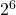, then 1 foretc until you get to the last two zeros – they are for noand.

Example 2

Let’s translate the base 2 number:to base 10.

We have to remember that the first place on the right is the number of, not.

Therefore the highest power of 2 in this example is. We have:## Short Comprehension Exercise:

1. What is the numberin base 10?
2. What is the numberin base 10?
3. Write the number 55 in base 2.
4. Write the year you were born in base 2.

# Goal – writing numbers in base 16

When writing in base 16, we use the digitsalong with powers of 16.

Now the problem is, the numbersare all two digit which can get confusing. So we have a genius plan of giving those numbers letter names. Instead of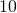, we write ‘A’, instead of, we write ‘B’ etc. Therefore, in base 16 we use the ‘digits’:Example 1: Let’s write the base 10 number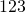in base 16.

Now we can’t get aout of, becauseis too high. We’ll have to figure out how manywe get, and what’s left.

Now, and leaves us with 11 left. So we have: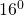0 7 11

We have.

Therefore the number 123 is equal to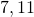in base 16, or better:.

Example 2: The colour #FA 80 72 is also known as ‘salmon’. Let’s translate it to base 10 to find the Red, Green, Blue parts.

The Red part is [F A]. Now F stands for 15; A stands for 10. So we have.

The Green part is [8 0]. That is,.

The Blue part is [7 2]. That is,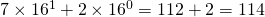Click here to type in those three values: 250 Red; 128 Yellow; 114 Blue, to see the colour!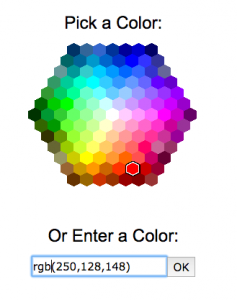# Back to the Project

Now that you can translate the # numbers for colours, go ahead and swap your colour wheel design with another group. Translate their code, and reproduce their colour wheel.